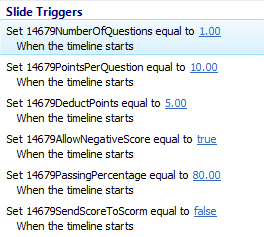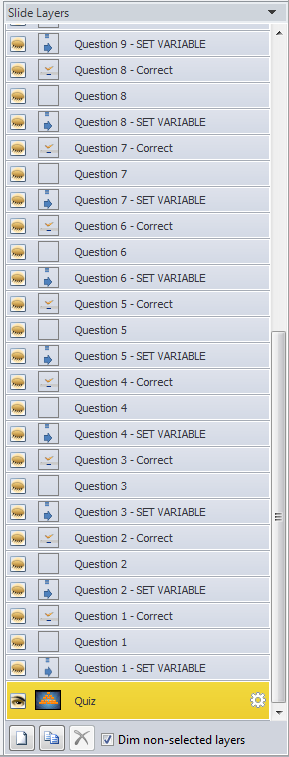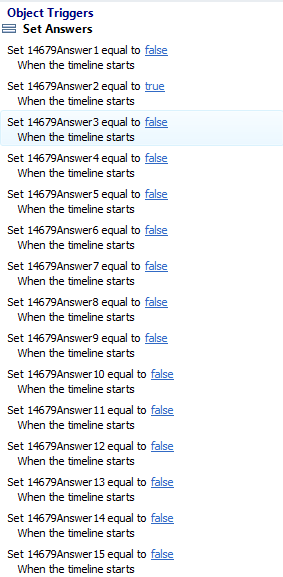# How to use the Pyramid Storyline Game

Pyramid Storyline Game

This game is composed of 3 different slides. The 'Introduction Slide' (1st slide), 'Quiz Slide' (2nd slide), and 'Results Slide' (3rd slide). IN the 2nd slide you with find the quiz questions. For each question in the game there is a 'Slide Triggers' layer, which is where most of the setting for this game are at. In this tutorial I will show you how to set the:

• Number of Questions,
• Points Per Question,
• Deduction Points,
• Other features for this game.On the "Slide Triggers" window that's on the 1st Slide, you can set this following variables:

1. 14679NumberOfQuestions

- This variable will allow you to set your desired number of questions you want to have. 1-15 Questions.

2. 14679PointsPerQuestion

- This variable will allow you to set the correct points per question.

3. 14679DeductPoints

-  This variable will allow you to set the deduction points per incorrect answer.

4. 14679AllowNegativeScore

- This variable will allow you to set if you want this game to have a negative score. Set this to true if you allow to have a negative score or otherwise, false.

5. 14679PassingPercentage

- This variable is use for the Passing Percentage Score in the LMS. If the Learner's score is greater or equal to this variable, it will report to the LMS as passed. Otherwise, failed.

6. 14679SendScoreToScorm

This variable allows this game to report score in LMS. Set this to true if you want to publish this game in to LMS or otherwise, false.

On the second slide, this is where you can set your answers for each question. If  you look into Slide Layers, go to Question 1 - SET VARIABLE layer to set the Question 1 Correct Answers. Question 2 - SET VARIABLE layer to set the Question 2 Correct Answers and so on.Each true or false Variable (14679Answer) is set up to reflect the answer choices in the game. So, typically for Question 1 the correct answer is answer choice 1. You would then want to have 14679Answer1 = True. However, this really comes in handy when a question will have multiple correct answers. So, say for question 2 answer choice 2, 3, and 4 are correct and if the learner selects either one of them they get the question right. All you need to do is set 14679Answer2, 3, and 4 = True. Most likely for Questions 3 and 4 those same answer choices will be correct for them as well, so have those same variable be true for those questions also.

Clear as mud? I hope you find value in this tutorial and like the game just as much as I do. Good luck and happy eLearning!